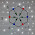Lewis Dot Structures - Simple Procedure for writing Lewis Structures of Nitrous Oxide (N2O) | Chemistry Net

# Lewis Dot Structures - Simple Procedure for writing Lewis Structures of Nitrous Oxide (N2O)

Lewis electron dot structure of nitrous oxide N2O

# Lewis Electron Dot Structure of N2O

A simple procedure for writing Lewis structures is given in a previous article entitled “Lewis Structures and the Octet Rule”. Several worked examples relevant to this procedure were given in previous posts please see the Sitemap - Table of Contents (Lewis Electron Dot Structures).

Another example  for writing Lewis structures following the above procedure is given below.

Let us consider the case of the dot structures of nitrous oxide N2O. Nitrous oxide is a colorless and odorless gas that is used as an anesthetic and analgesic in surgery and dentistry. It is also known as “laughing gas” due to the euphoric effects of inhaling it. It is used as an oxidizer in rocketry and in motor racing to increase the power output of engines. Nitrous oxide accounts for about 5% of the greenhouse  effect. Although its atmospheric concentration is only 320 ppb, it has 300 times greater ability to absorb infrared radiation than CO2 does. The resonance structures for N2O are as follows:

How can we construct the N2O Lewis structure?

Step 1: The central atom will be the N atom since it is the less electronegative. Connect the atoms with single bonds:

Step 2: Calculate the # of electrons in π bonds (multiple bonds) using  formula (1) in the article entitled “Lewis Structures and the Octet Rule”.

Where n in this case is 3 since N2O consists of three atoms.

Where V = (5 + 5 + 6 ) = 16

Therefore, P = 6n + 2 – V = 6 * 3 + 2 – 16 = 4

There are 4 π electrons in N2O.

Therefore, 2 double bonds or 1 triple bond must be added to the structure of Step 1.

Step 3 & 4: The Lewis structures for N2O are as follows:

Please watch also the following video regarding the Lewis structures of Nitrous oxide:

Relevant Posts - Relevant Videos

Lewis Structures|Octet Rule: A Simple Method to write Lewis Structures

Simple Method for writing Lewis Structures for N2O3

References

1. G.N. Lewis, J.A.C.S, 38, 762-785, (1916)
2. E. C. McGoran, J. Chem. Educ., 68, 19-23 (1991)
3. A.B.P. Lever, J. Chem. Educ., 49, 819-821, (1972)

Key Terms

resonance structures of nitrous oxide N2O, Lewis electron structures of nitrous oxide, chemical formula of N2O3, simple procedure for drawing Lewis structures of nitric acid,

1.Isn't the second Lewis structure the more plausible one? since O has a larger electronegativity than N...

1.Oxygen is more electronegative than nitrogen and therefore can accommodate better a negative charge. So you are correct Lewis structure #2 is the most plausible one. Thank you for your comment!

2.N2O on the basis of the three-electron bond.

Using three-electron bond one can explain structure of N2O, carbonate anion, carboxylate anion, oxygen molecule, structure of nitrate anion, nitrogen monoxide, ozone, naphthalene and other organic and non-organic compounds.

It is easy to show, that relation multiplicity = f(L) and Е = f(L), where multiplicity is multiplicity of bond, L – length of bond in Å, Е – energy of bond in kj/mole will be described by function y = a + b/x + c/x² for any types of bond (C-N, C-O, C-S, N-N, N-O, O-O, C-P).

See link: A short analysis of chemical bonds http://vixra.org/pdf/1606.0149v2...

N2O:

N−N = 1.1282 Å
N−O = 1.1843 Å

Multiplicity (L N-N = 1.1282 Å) = 2.730
Multiplicity (L N-O = 1.1843 Å) = 1.969

p. 15 http://vixra.org/pdf/1606.0149v2.pdf

http://vixra.org/author/bezverkhniy_volodymyr_dmytrovych

http://vixra.org/pdf/1612.0018v1.pdf

1.See link: A short analysis of chemical bonds http://vixra.org/pdf/1606.0149v2.pdf